Since this blog is tailored for beginners in econometrics, I will not be engaging an advanced discussion on the topic but an introductory approach by which a beginner can understand the essence of using lags in a model and the pitfalls that may occur if lags are excessively used. Interested readers who require advanced information on selecting optimal lags can consult appropriate econometric textbooks. Having said that, in economics the dependence of a variable Y (outcome variable or regressand) on another variable(s) X (the predictor variable or regressor) is rarely instantaneous. Very often, Y responds to X with a lapse of time. Such a lapse of time is called a lag. Therefore, in time series analysis, some level of care must be exercised when including lags in a model.

So how many lags should be used in a model? There is no hard-and-fast-rule on the choice of lag length. It is basically an empirical issue. As noted in Damodar Gujarati Basic Econometrics, there is no a priori guide as to what the maximum length of the lag should be. The researcher must bear in mind that, as one estimates successive lags, there are fewer degrees of freedom left, making statistical inference somewhat unstable. Economists are usually not that lucky to have a long series of data so that they can go on estimating numerous lags. More importantly, in economic time series data, successive values (lags) tend to be highly correlated increasing the likelihood of multicollinearity in the model.

Also, from Jeffery Wooldridge’s Introductory Econometrics: A Modern Approach with annual data, the number of lags is typically small, 1 or 2 lags in order not to lose degrees of freedom. With quarterly data, 1 to 8 lags is appropriate, and for monthly data, 6, 12 or 24 lags can be used given sufficient data points. Again, in the words of Damodar Gujarati Basic Econometrics “the sequential search for the lag length opens the researcher to the charge of data mining”. He further stated that the nominal and true level of significance to test statistical hypotheses becomes an important issue in such sequential searches”. For instance, if the lag length, k, is incorrectly specified, the researcher will have to contend with the problem of misspecification errors. In addition, because of the lags involved, distributed and or autoregressive models raise the topic of causality in economic variables.

Hence, before you estimate a time series equation, it is necessary to decide on the maximum lag length. Like I mentioned earlier, this is purely an empirical question. Suppose there are 40 observations in all, by including too many lagged values, your model consumes degrees of freedom, not to mention introducing the likelihood of multicollinearity occurring. As noted in my previous tutorial on multicollinearity, it leads to imprecise estimation; that is, the standard errors tend to be inflated in relation to the estimated coefficients. As a result, based on the routinely computed t ratios, we may tend to declare (erroneously), that a lagged coefficient(s) is statistically insignificant. In the same vein, including too few lags will lead to specification errors. The easiest way out of this quagmire, is to decide using a criterion like the Akaike or Schwarz and choose that model that gives the lowest values of these criteria. Most econometric packages easily compute these optimal lag length but note some trial and error is inevitable.

Choosing Optimal Lags in Stata

For this tutorial, I will extract data from Gujarati and Porter Table 21.1 dataset. It is a quarterly data on United States from 1970 to 1991, which is 88 observations. The variables are gdp (gross domestic product), pdi (personal disposable income) and pce (personal consumption expenditure).

Step 1: Load data into Stata
Step 2: Prepare Stata for Analysis
Inform Stata that you are about to perform a time series analysis by typing this code into the Command box: tsset qtrly

and you will obtain this response: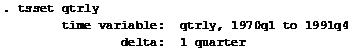Stata - tsset Command Source: CrunchEconometrix
Stata now recognises that you are about conducting a time series analysis using quarterly data from 1st quarter of 1970 to the 4th quarter of 1991. If you don’t issue this command, Stata will not run your analysis.

Step 3: Obtain Model Lag Length
Type this code into the Command box: varsoc gdp pce pdi

…and the Stata output for the model (shown below) indicates that lag 2 is the optimal lag and that AIC is the best criterion for the model given it has the lowest value, 26.8144.Stata - Optimal Lags for the Model Source: CrunchEconometrix

Step 4: Obtain Variables Lag Length
Optimal lags can be obtained for the respective variables and the rule-of-thumb remains the same. We select that lag identified by the criterion which gives the lowest value.

So, for gdp, type this code into the Command box: varsoc gdp

…and the Stata output indicates that the optimal lag length for gdp is 2.Stata - Optimal Lags for gdp Source: CrunchEconometrix

To obtain optimal lag for pce, type: varsoc pce

…Stata output indicates that the optimal lag length for pce is 4.Stata - Optimal Lags for pce Source: CrunchEconometrix

varsoc pdi
…Stata output indicates that the optimal lag length for pdi is 1.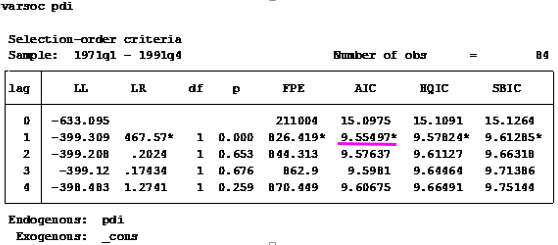Stata - Optimal Lags for pdi Source: CrunchEconometrix

Caveat: There are also cases where the used lag length is that which is most selected by the criterion named after the econometricians who developed them, like HQ, SIC, AIC and LR, etc. Some researchers prefer Schwartz criterion when the variables are more than 4 and use the AIC when the variables are less than 4. As, mentioned in the introductory part of this tutorial, the decision on the choice of lag is purely an empirical issue. Generally, we choose the lag length for which the values of most of these lag length criteria are minimized, indicated by asterisks in the EViews output.

[Watch video tutorial on lag selection using Stata]

Having gone through this tutorial, it will be easy for you to determine the optimal lag for your model regardless of the analytical package used. The basics are the same. Remember that “Lag length criteria” indicate a definite way of selecting the optimal lag after estimating the initial VAR model (in EViews). Also VAR and ARDL models are susceptible to arbitrary use of lags as this may erode the degrees of freedom, weaken the significance of the coefficients, may induce auto-correlation and weaken the strength of diagnostic tests.

Try these steps on your models and if there are further and comments, do post them below…..

How to Interpret Regression Output in Excel

The dissertation buzz is on and students are doing everything possible to meet up with the deadline. This is the current atmosphere in tertiary institutions, at least for those with undisrupted academic calendar J. The students are in different stages of their project, as it is commonly called. Some are yet to wrap up their chapter one which gives the “study background” and the framing of research hypotheses, objectives and questions. Some have moved on to chapter two reviewing relevant literature related to their scope of study. Others have gone further in developing both the theoretical and empirical frameworks for chapter three, but not without the usual teething lags…but they’ll get around it, somehow J. A handful have even done better by progressing to chapter four attempting to analyse their data.

Since, chapters one to three are relative to each students’ scope of research, however, a regression output is common to all (although actual outcomes differ). It is based on this that I decided to do a tutorial in explaining the basic features in a regression output. Likewise, this write-up is in response to requests received from readers on (1) what some specific figures in a regression output are and (2) how to interpret their results. Let me state here that regardless of the analytical software whether Stata, EViews, SPSS, R, Python, Excel etc. what you obtain in a regression output is common to all analytical packages (except where slight variations occur).

For instance, in undertaking an ordinary least squares (OLS) estimation using any of these applications, the regression output will churn out the ANOVA (analysis of variance) table, F-statistic, R-squared, prob-values, coefficient, standard error, t-statistic, degrees of freedom, 95% confidence interval and so on. These are the features of a regression output. However, the issue is: what do these mean and how can they be interpreted and related to a research.

Hence, the essence of this tutorial is to teach students the relevance of these features and how to interpret their results. I will be using Excel analytical package to explain a regression output, but you can practise along using any analytical package of your choice. (See tutorial for Stata and EViews users).

An Example: Use Gujarati and Porter Table7_12.dta or Table7_12.xlsx dataset
Note: I will not be discussing stationarity or cointegration analysis in this tutorial (that will come later on). Since the issue on how to understand the features of a regression output and interpret results, I will just be doing a simple linear regression analysis (a bi-variate analysis) with only one explanatory variable.

The dataset is on the United States from 1960 to 2009 (50 years data). The outcome variable is consumption expenditure (pce) and the explanatory variable is income (income).

Before you begin, ensure that the DATA ANALYSIS Add-in is in your tool bar because without it, you cannot perform any regression analysis. To obtain it follow this guide:
File >> Options >> Add-ins >> Excel Options dialog box opens
Under Active Application Add-ins, choose Analysis ToolPak
In the Manage section, choose Excel Add-ins
Click Go, then OK

If it is correctly done, you should see this:Excel Add-in Dialog Box Source: CrunchEconometrix

Here is the data in excel format:

Third step: Visualise the relationship between the variables
Before analysing the data, it is good to always graph the dependent and key explanatory variable (using a scatter plot) in order to observe the pattern between them. This gives you what to expect in your actual analysis. Here’s the procedure:
1.    Highlight the 2 columns that contain the variables
2.    Go to Insert >> Charts >> Scatter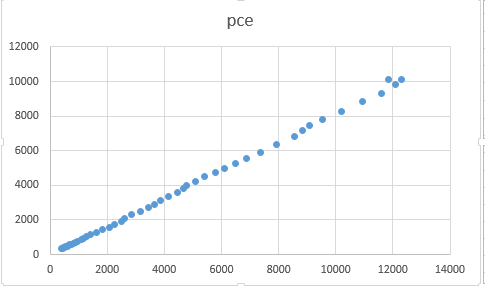Excel - Scatter plot of pce and income Source: CrunchEconometrix

The graph indicates a positive relationship between the two variables. This seems plausible because the higher your income, the higher will be your consumption, except you are very frugalJ.

The graph can be formatted by adding a trend line (see video on how to do this). In Excel, adding a trendline also gives you the linear prediction:

As can be seen from the second graph, we have the linear prediction for pce and the R2.

Fourth step: The scientific investigation
Now we want to scientifically investigate the relationship between pce and income. To do this, go to Data >> Data Analysis (dialogue box opens) >> Regression >> OK.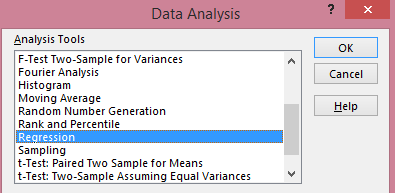Excel - Data Analysis Dialogue Box Source: Crunch Econometrix
Once you click OK, the Regression dialogue box opens: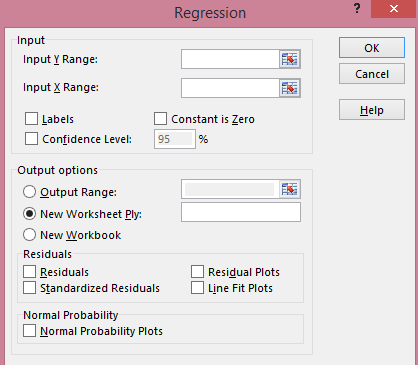Excel - Regression Dialogue Box Source: CrunchEconometrix

·      Put data range for pce under Input Y Range
·      Put data range for income under Input X Range
·      Check label box
·      Check Confidence Level box
·      Check Output range
·      Click OK
(You have simply told Excel to regress the dependent variable, pce, on the explanatory variable, income), and the output is shown as:

Fifth step: The features of a regression output
The Excel output gives the Regression Statistics and the ANOVA table. So what do these figures mean? I will explain each feature in turns.

Under “Regression Statistics”:
R-squared: gives the variation in pce that is explained by income. The higher the R2, the better the model and the more predictive power the variables have. Although, an R2 that equals 1 will elicit some suspicion. The R is actually the correlation coefficient between the 2 variables. This implies that
= the correlation coefficient.

Standard Error: this is the standard error of the regression

Observations: the data span is from 1960 to 2009 = 50 years

Under “ANOVA” (analysis of variance):
Source: there are two sources of variation on the dependent variable, pce. Those explained by the regression (i.e, the Model) and those due to randomness (Residuals)

df: this is degree of freedom calculated as k-1 (for the model) and n-k (for the residuals)

SS: implies sum of squared residuals for the Regression (explained variation in pce) and Residuals (unexplained variation in pce). After doing the regression analysis, all the points on pce do not fall on the predicted line. Those points outside the line are known as residuals. Those that can be explained by the regression are known as Explained Sum of Squares (ESS) while those that are due to random forces, which are outside the model are known as Residual Sum of Squares (RSS).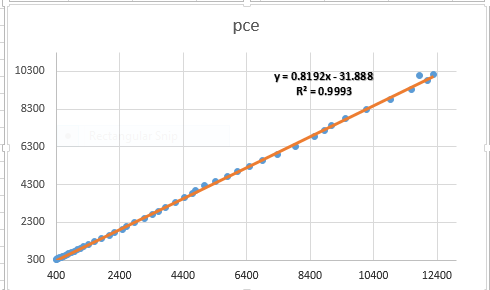Excel - Predicted Value of pce Source: CrunchEconomterix
As observed from the graph, all the points do not fall on the predicted line. Some lie above, while some are beneath the line. These are all the residuals (in order words, the remnants obtained after the regression analysis). If the predicted line falls above a point, it means that pce is over-predicted (that is, pce – pcehat is negative) and if it is beneath a point, it implies that pce is under-predicted (that is, pce – pcehat is positive). The sum and mean of the residuals equals zero.

MS: implies mean sum of squared residuals obtained by dividing SS by df i.e. SS/df

F: captures whether the explanatory variable, income is significant in explaining the outcome variable, pce. The higher the F-stat, the better for the model.

Significance F: this is the probability value that indicates the statistical significance of the F ratio. A significance-value that is less than 0.05 is often preferred.

Coefficient: this is the slope coefficient. The estimate for income. The sign of the coefficient also tells you the direction of the relationship. A positive (negative) sign implies a positive (negative) relationship.

Intercept: this is the hypothetical outcome on pce if income is zero. It is also the intercept for the model.

Standard error: this is the standard deviation for the coefficient. That is, since you are not so sure about the exact value for income, there will be some variation in the prediction for the coefficient. Therefore, the standard error shows how much deviation occurs from predicting the slope coefficient estimate.

t-stat: this measures the number of standard errors that the coefficient is from zero. It is obtained by: coeff/std. error. A t-stat above 2 is sufficient evidence against the null hypothesis

P-value: there are several interpretations for this. (1) it is smallest evidence required to reject the null hypothesis, (2) it is the probability that one would have obtained the slope coefficient value from the data if the actual slope coefficient is zero, (3) the p-value looks up the t-stat table using the degree of freedom (df) to show the number of standard errors the coefficient is from zero, (4) tells whether the relationship is significant or not.

So, if the p-value is 0.3, then it means that you are only 70% (that is, (100-30)% ) confident that the slope coefficient is non-zero. This is not good enough. This is because a very low p-value gives a higher level of confidence in rejecting the null hypothesis. Hence, a p-value of 0.02, implies that you are 98% (that is, (100 - 2)% ) confident that the slope coefficient is non-zero which is more re-assuring! J.

Lower and Upper 95%: these are the confidence intervals. If the coefficient is significant, this interval will contain that slope coefficient but it will not, if otherwise.

Assignment:
Use Gujarati and Porter Table7_12.dta or Table7_12.xlsx dataset.
(1)  With pce as the dependent variable and gdpi as the explanatory variable, plot the graph of pce and gdpi, what do you observe?
(2)  Run your regression. Can you interpret the table and the features?
(3)  Plot the predicted line. What are your observations?

I have taken you through the basic features of a regression output using Excel data analysis software on ordinary least squares (OLS) model in a simple linear regression. So, you now have the basic idea of what the F-stat, t-stat, df, SS, MS, prob>F, p>|t|, confidence interval, R2, coefficient, standard error stand for.

[Watch video on "How to interpret regression output in Excel"]

Practice the assignment and if you still have further questions, kindly post them below....

Since this blog is tailored for beginners in econometrics, I will not be engaging an advanced discussion on the topic but an introductory approach by which a beginner can understand the essence of using lags in a model and the pitfalls that may occur if lags are excessively used. Interested readers who require more advanced information on lag selection can consult appropriate econometric textbooks. Having said that, in economics the dependence of a variable Y (outcome variable or regressand) on another variable(s) X (the predictor variable or regressor) is rarely instantaneous. Very often, Y responds to X with a lapse of time. Such a lapse of time is called a lag. Therefore, in time series analysis, some level of care must be exercised when including lags in a model.

So how many lags should be used in a model? There is no hard-and-fast-rule on the choice of lag length. It is basically an empirical issue. As noted in Damodar Gujarati Basic Econometrics, there is no a priori guide as to what the maximum length of the lag should be. The researcher must bear in mind that, as one estimates successive lags, there are fewer degrees of freedom left, making statistical inference somewhat unstable. Economists are usually not that lucky to have a long series of data so that they can go on estimating numerous lags. More importantly, in economic time series data, successive values (lags) tend to be highly correlated increasing the likelihood of multicollinearity in the model.

Also, from Jeffery Wooldridge’s Introductory Econometrics: A Modern Approach with annual data, the number of lags is typically small, 1 or 2 lags in order not to lose degrees of freedom. With quarterly data, 1 to 8 lags is appropriate, and for monthly data, 6, 12 or 24 lags can be used given sufficient data points. Again, in the words of Damodar Gujarati Basic Econometrics “the sequential search for the lag length opens the researcher to the charge of data mining”. He further stated that the nominal and true level of significance to test statistical hypotheses becomes an important issue in such sequential searches”. For instance, if the lag length, k, is incorrectly specified, the researcher will have to contend with the problem of misspecification errors. In addition, because of the lags involved, distributed and or autoregressive models raise the topic of causality in economic variables.

Hence, before you estimate a time series equation, it is necessary to decide on the maximum lag length. Like I mentioned earlier, this is purely an empirical question. Suppose there are 40 observations in all, by including too many lagged values, your model consumes degrees of freedom, not to mention introducing the likelihood of multicollinearity occurring. As noted in my previous tutorial on multicollinearity, it leads to imprecise estimation; that is, the standard errors tend to be inflated in relation to the estimated coefficients. As a result, based on the routinely computed t ratios, we may tend to declare (erroneously), that a lagged coefficient(s) is statistically insignificant. In the same vein, including too few lags will lead to specification errors. The easiest way out of this quagmire, is to decide using a criterion like the Akaike or Schwarz and choose that model that gives the lowest values of these criteria. Most econometric packages easily compute these optimal lag length but note some trial and error is inevitable.

Choosing Optimal Lags in EViews

For instance, if there are limited observations in a vector autoregressive (VAR) estimation, it is often advised to use the Akaike Selection Criterion (AIC) in selecting the lag length that "prefers" the more parsimonious models. However, the information criterion with the smallest criterion value evidences the most ideal lag length to employ. Most researchers prefer using the Akaike information criterion (AIC) but my valuable advice is always to select that criterion with the smallest value, because that ensures the model will be stable. Let us begin by showing how you can select the optimal lag order for your model and variables using the EViews analytical package.

Please note that in EViews, the procedure is simply to run an initial VAR on the variables at level with the default settings and obtain the results. I will go through the steps in detail.

For this tutorial, I will extract data from Gujarati and Porter Table 21.1 dataset. It is a quarterly data on United States from 1970 to 1991, which is 88 observations. The variables are gdp (gross domestic product), pdi (personal disposable income) and pce (personal consumption expenditure).

Step 1: Load Data into EViews
To import the Excel file into EViews, go to: File >> Import >> Import from file >> Next >> Finish. If it is correctly done, you obtain:EViews Workfile Source: CrunchEconometrix
From the EViews interface, the three variables gdp, pce and pdi are individually shown. Double-clicking on each variable shows them in separate sheets, like is:EViews Creating Group Data Source: CrunchEconometrix

Step 2: Create Group Data
But because I need to obtain the optimal lag for the model, it becomes necessary to open this data as a GROUP by putting all three variables in a worksheet. To do that: Press down the Cntrl key >> click on gdp, pce and pdi >> Right click on any part of the screen >> Open >> as Group: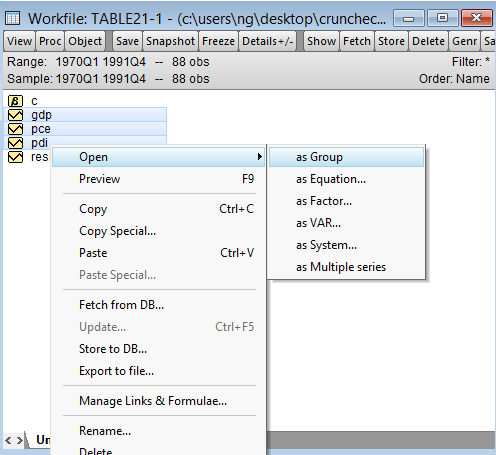EViews - Open as Group Data Source: CrunchEconometrix

When you click "as Group", you should have this:EViews Group Data Source: CrunchEconometrix

Step 3: Run Unrestricted VAR model
Now that our variables are grouped, next is to run an unrestricted VAR model with the level of the variables and taking different lags before deciding which model is the best. Remember, I am using quarterly data which allows me to use up to 8 lags. But if yours is a yearly data you can use 2 lags at the most in order not to lose too many degrees of freedom or if monthly data, up to 24 lags. The unrestricted VAR is chosen only on the assumption that the three variables are not co-integrated.

Note: if the variables are cointegrated, you should run the vector error correction model

To run the unrestricted VAR model, go to: Quick >> Estimate VAR >> Dialog box opens:EViews VAR Specification Source: CrunchEconometrix

Type in all the variables names in the Endogenous variables box (note under VAR, there is no exogenous variable, all variables are endogenous). Since between 1 to 8 lags can be used because I am using a quarterly data, I begin with 4 lags before deciding which model is the best.

Click OK….here is the output (to save space only relevant part shown):EViews Regression Output Source: CrunchEconometrix
The EViews output reports among others, the AIC and Schwarz criterion. You will also observe that the output returned 2 sets of results, those identified by red bracket are for the respective endogenous variables with each column representing the result for gdp, pce and pdi in that order. But the results we are most interested in are those identified by the blue bracket. These are the estimates for the VAR system. However, at this moment, we are only interested in the criterion. Hence, between the AIC and Schwartz, the former’s criterion of 26.85144 is lower than that of Schwartz at 27.98004. Therefore, we conclude based on this output that the lag selection must be based on the AIC.

Step 4: Choose Optimal Lag length for the Model
However, we cannot be running the unrestricted VAR model using different lag lengths before deciding on the best model to adopt, there is a simplified way of obtaining the optimal lag structure at once given a variety of information criteria. To do that, click on View >> Lag Structure >> Lag Length Criteria >> the Lag Specification dialog box opens:EViews Lag Specification Dialogue Box Source:CrunchEconometrix
Note: I put in 8 lags because I am at liberty to use up to 8 lags due to the nature of my data (quarterly). So, if yours is a yearly data, you may put in 2.

Click OK to obtain the various information criterion from lag 0 to 8 shown below: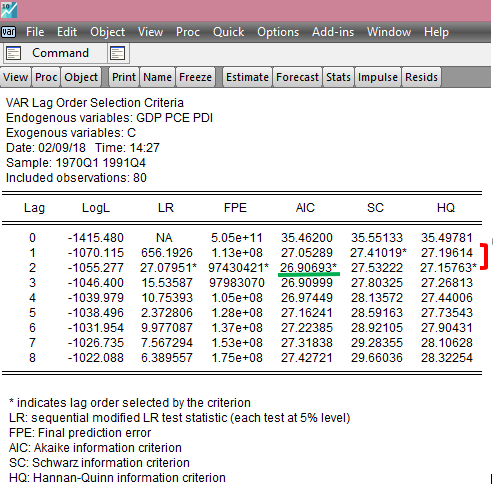EViews Model Lag Structure Source: CrunchEconometrix
From the output, the selected lag order is indicated by an asterisk sign (*) which is distributed between lags 1 and 2, but mostly on lag order 2. The rule-of-thumb is to select the criterion with the lowest value which again is the AIC at 26.90693 this is because the lower the value, the better the model. We can conclude that the optimal lag length for the model is 2 and the best criterion to adopt for the model is AIC.

The same procedure can be adopted in obtaining the respective lags for each variable. For instance to obtain for gdp:
1.   Double click on gdp >> Quick >> Run the unrestricted VAR >> OK >> Obtain the output
2.   Click View >> Lag Structure >> Lag Length Criteria >> Lag Specification dialog box opens >> OK

…and you obtain this: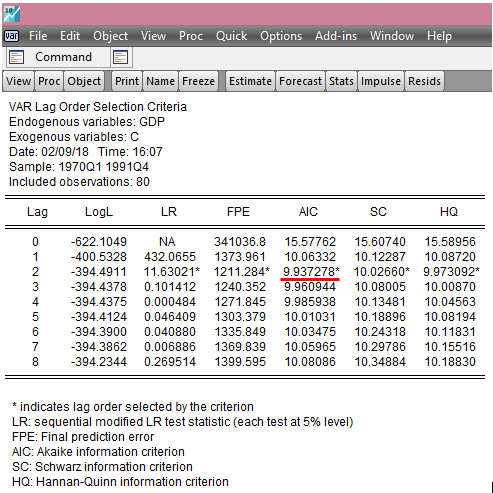EViews - Lag Structure for gdp Source: CrunchEconometrix

From the output, the best criterion that fits the gdp model is the AIC with the lowest figure of 9.937278 meaning that the optimal lag length for gdp is 2.

Doing the same procedure for pce, here is the result:EViews - Lag Structure for pce Source: CrunchEconometrix
From the output, the optimal lag length for pce model is 4 given the AIC value at 8.698617 which the lowest among the criterion, hence it is the best criterion for the pce model. For pdi, the optimal lag length is 1 given the AIC value at 9.602079 shown below: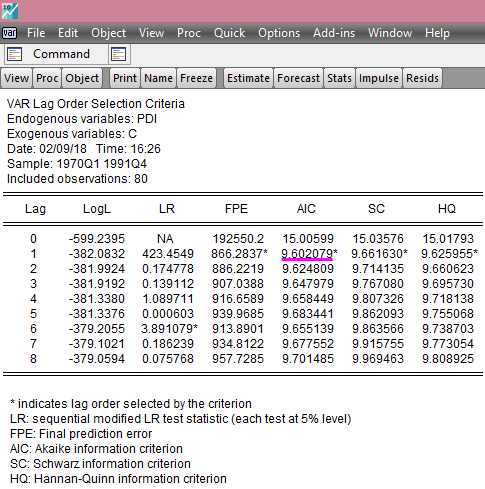EViews - Lag Structure for pdi Source: CrunchEconometrix
Caveat: There are also cases where the used lag length is that which is most selected by the criterion named after the econometricians who developed them, like HQ, SIC, AIC and LR, etc. Some researchers prefer Schwartz criterion when the variables are more than 4 and use the AIC when the variables are less than 4. As, mentioned in the introductory part of this tutorial, the decision on the choice of lag is purely an empirical issue. Generally, we choose the lag length for which the values of most of these lag length criteria are minimised, indicated by asterisks in the EViews output.

[Watch video tutorial on optimal lag selection using EViews]

Having gone through this tutorial, it will be easy to understand and know how to determine the optimal lags for a model regardless of the analytical package used. Remember that the “Lag length criteria” indicates a definite way of selecting the optimal lags after estimating the initial VAR model. Also VAR and ARDL models are susceptible to arbitrary use of lags as this may erode the degrees of freedom, weaken the significance of the coefficients, may induce auto-correlation and weaken the strength of diagnostic tests.

Try the outlined steps on your models and if there are further and comments, do post them below…..